• Hash256
• Hash160
• Reverse Bytes
• Satoshis

# Public Keys

A unique number generated from your private key.

## What is a public key?

A public key is the early form of an address.

And like a private key, it’s stored as a big hexadecimal value:

If we didn’t end up compressing this public key in to a shorter format (called an address), this would have been the “account number” that you would have sent bitcoins too.

## How do you get a public key from a private key?

You insert the private key in to a special mathematical function, and it gives you a public key.

### What is this function?

It’s called elliptic curve multiplication, which basically involves “bouncing around a graph of an elliptic curve” until you end up at a final co-ordinate on the graph. And the numbers of this final co-ordinate give you your public key.

It’ll be easier if I show you…

### What does an elliptic curve look like?

Like this:

Furthermore, the elliptic curve used in bitcoin comes with a specific starting point.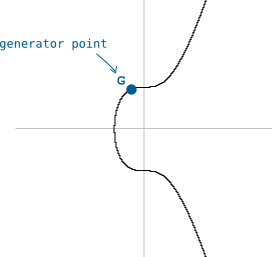And if we were to do some basic “multiplication” on this curve (e.g. “multiplying” the starting point by 2), we would move around the curve like this.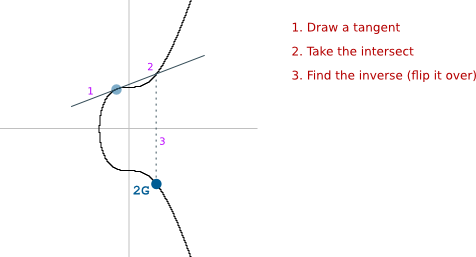The fact that we can draw a tangent anywhere on the curve and it will intersect one other point on the curve is a special feature of elliptic curves.

And there we have it. We have just “multiplied” the starting co-ordinate (G) by 2, and found the position of the final co-ordinate (2G).

This is one round of elliptic curve multiplication.

I keep putting “multiplication” inside quotes because it’s not standard multiplication as you know it.

If you were to multiply the co-ordinates of G by 2, it would not give you the co-ordinates of 2G (as shown on the graph).

You see, the geniuses who found out that you can move around the curve in this way had to call it something, so they to decided refer to it as “multiplication”.

Because, you know, mathematics can never be confusing enough.

When I say “multiplication” from now on, I mean “elliptic curve multiplication”.

### How do you get a public key?

In the above example we multiplied `G` by 2 to get `2G`.

To get a public key, we multiply G by our private key.

Or in other words, “bounce around the elliptic curve private key number of times.”

The final resting point on the elliptic curve will give you a set of co-ordinates, and these co-ordinates form your public key.

So if these are the co-ordinates we end up with after multiplying `G` by our `private key`:

Then all we have to do is convert both to hexadecimal and smush them together…

This is the original format of public key, which means I’ve got to put an `04` at the start. Like this:

To find out why this is the case, I’m afraid you’re going to have to have a read through the section about compressed public keys

And ta da! A public key!

God almighty.

### Compressed Public Keys

To save space, public keys (these days) only use the full `x` co-ordinate.

This is because the elliptic curve is an equation (`y2 = x3 + 7`), which means that if you have the `x` co-ordinate, you can still work out the corresponding `y` co-ordinate.

However, due to the `y2` part of the equation, the `y` could be a positive or negative number: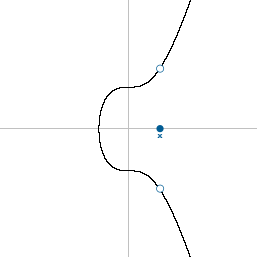So the only extra information you need to find the correct y co-ordinate is to know whether the y co-ordinate is above or below the x-axis. And due to the way elliptic curves work:

• If y is even, it’s above the x-axis.
• If y is odd, it’s below the x-axis.

So instead of having to store both `x` and `y` as the public key, you can just store `x` and whether y is (`even` or `odd`).

In bitcoin, the even or odd part is shown by prepending a number to the x co-ordinate.

• `even = 02`
• `odd = 03`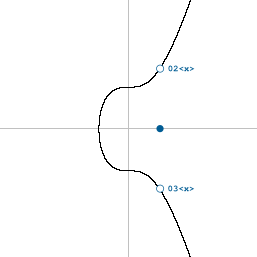So whereas an original public key will begin with `04`, a compressed public key will begin with either `02` or `03`:

This seems like a lot of effort for a little less text, but because public keys are used within transaction data, it does end up saving a lot of space in the blockchain over time.

## Why do we use elliptic curve multiplication to make public keys?

Because elliptic curves have two properties that are useful when it comes to creating a private/public key pair.

1. Elliptic curve multiplication is a “trapdoor function”. In other words, you can’t go backwards (i.e. “divide” the co-ordinates of a public key) to find out what the private key was.

A trapdoor function is a function that is easy to compute in one direction, yet difficult to compute in the opposite direction (finding its inverse) without special information, called the “trapdoor”.

1. Nonetheless, the public key is still mathematically connected to the private key. As a result, it’s possible to prove this connection (with a little more mathematics) without having to reveal your private key.

So if I gave you my public key (or address), I can prove to you that I “own” it without having to show you my private key.

This ability is especially handy when I want to make a bitcoin transaction, which involves putting my public key in to the transaction data and proving that I’m the owner of it, without having to put my private key in to the transaction data too.

A public key is a unique number that has been created from a private key. So when I say “prove I own” a public key, I mean “show that I know the number of the private key that the public key was created from”.

### How can you prove you own a public key?

As mentioned, there’s a mathematical connection between my private key and my public key.

As a result:

1. I can put my private key through some more elliptic curve mathematics to get a new value.
2. I can put my public key through some other elliptic curve mathematics to get another new value.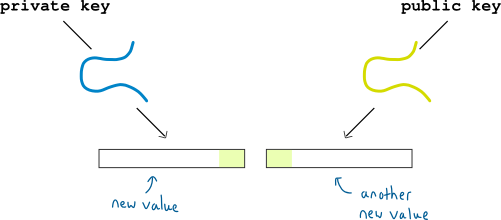Now, there will be some small overlap in these new values.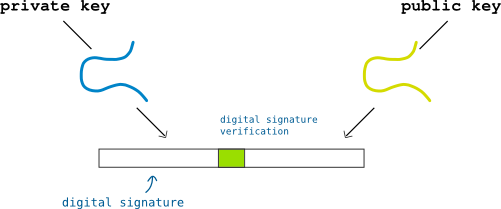The new value I can create from my private key is called a digital signature

And…

The overlap proves that there is a mathematical connection between the public and private key.

And because nobody is able to recreate this digital signature without the private key, my digital signature it’s enough to prove that I “own” the public key.

So I can show you that I own a public key with a digital signature, and you never need to see my private key.

### Conclusion.

All hail the elliptic curve.

Hey there, it's Greg.

I'll let you know about cool website updates, or if something seriously interesting happens in bitcoin.

Don't worry, it doesn't happen very often.